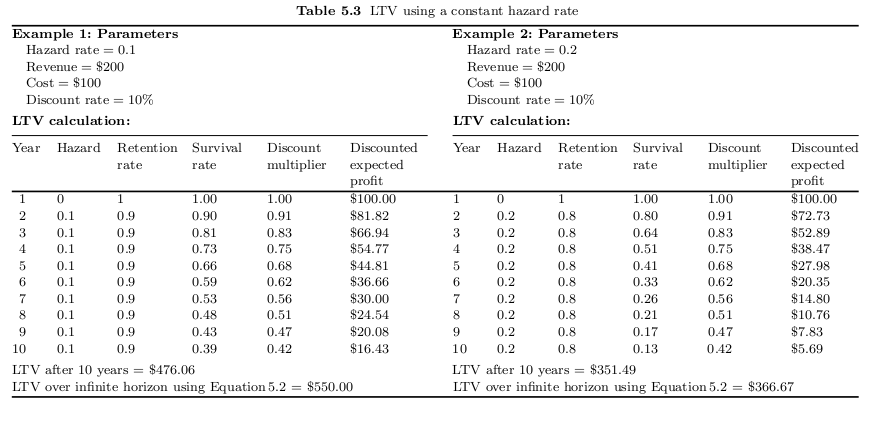### Introduction

This paper aims to layout the current state of Customer Lifetime Value calculation research. It is entirely practical, so mathematical descriptions will only be discussed where they are important from a practical perspective. It also aims to provide both code and spreadsheets to allow for usage of the models discussed. None of the work shown and discussed here is original, I will point out references in the text but please also see the references listed in the bibliography for further details.

### List of Models:

##### Contractual
• Naive
• Recency Frequency Monetary (RFM) Summaries
• Markov Chains
• Hazard Functions
• Survival Regression
• Supervised Machine Learning using Random Forest

#### Non-Contractual

• Management Heuristics
• Distribution Based Approaches

#### CLV approaches

CLV research aims to model the net revenue that can be obtained from the behaviour of clients. Successfully answering this question allows for the reshaping of firm’s activites to maximise the profit that can be gained via:

-Adjusting the marketing expenditure so it is not wasted on net-negative cohorts.

-Targeting marketing expenditure to avoid a client leaving (Churning).

-Targeting former clients to restart profitable relationships.

CLV models tend to be in two forms; contractual vs uncontractual. Contractual tends to be easier to deal with as there is certainty of the clients current status (Alive vs. Dead). We will look in this paper at contractual accounts before moving onto the more difficult extensions developed for non-contractual relationships.

#### Basic Equation

The general idea of Customer Lifetime Value is easily stated:

“The net present value of the profits linked to a specific customer once the customer has been acquired, after subtracting incremental costs associated with marketing, selling, production and servicing over the customer’s lifetime.” (Blattberg et al. 2008)

Formally this can be written as:

$CLV = \sum\limits_{t=1}^\infty \frac{E[\hat{V}_t]}{(1+\delta)^{t-1}}$

(Taken from Blattberg et al. 2008)

Where the $$\hat{V}$$ represents the customer’s net profit to the firm and $$\delta$$ represents the discount rate at each time period.

A simple way of calculating this requires:

1. The probability of a client leaving in a given period. (t)
2. The discount multiplier of capital for the given period. (DR)
3. Expected Revenue per client.
4. Cost of relationship maintenance.

If point 1 (called “A”" below) is taken as stable throughout the lifespan it can be calculated for a given period as:

$\textbf{Survival Rate in Year t} = (1-A)^{t-1}$

Assuming a stationary discount rate (DR) we can calculate the discount rate for a given period it as:

$\textbf{Discount Multiplier} = 1/(1 + DR)^{t-1}$

This is trivial to produce in Excel and the output would look something like this:

# Model 1: Naive(Taken from Blattberg et al. 2008 p.110)

So we will now move onto the practical points regarding the collection of the data that we need.

###### From Historical Data

The least sophisticated approach to working out a model life time would be to merely take the proportion who leave duration a duration (lets call it A for attrition) and treat it as constant and universal. This then can be plugged in to the LTV equations above with the discount rate. Next we would take the average spend by all customers under investigation and plug this into the above equation. We can then model the future!